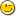### Re: Algebra problem

```Derek Parnell wrote:

> Erik-Jan van Kampen wrote:
>
>> Derek Parnell wrote:
>>>
>>> My high school algebra has deserted me>>>
>>>  Given the formula
>>>
>>>    A^b + Cb = d
>>>
>>> how do I solve for 'b'?
>>>
>>> --
>>> Derek Parnell
>>> Melbourne, Australia
>>>
>>
>> Hi Derek,
>>
>>  From the Matlab 6.5 Symbolic Toolbox:
>>
>>>> solve(A^b+C*b-d,b)
>>
>> ans =
>>
>> -(lambertw(log(A)/C*exp(d*log(A)/C))*C-d*log(A))/log(A)/C
>>
>
> Thanks. I thought it would be something simple like that ;-0
>
> This solution also implies that 'A' must > 1 and 'C' must be > 0
> otherwise there is no valid value for 'b'.

No generally.
A must not be = 1, but it can be < 1, e.g. for
A = 0.6
C = 3
d = 5

there are the two solutions:
b1 =  1.512753283
b2 = -6.184691234

```
```sequence s
atom A, C, d, b

A = 0.6
C = 3
d = 5
s = {1.512753283, -6.184691234}

-- check results
for i = 1 to length(s) do
b = s[i]
printf(1, "%f^%f + %f*%f = %f (expected: %f)\n", {A,b,C,b,power(A,b)+C*b,d})
end for
```

```

Also e.g.
A =  2
C = -3
d =  5

works for me.

Not only positive arguments are allowed for the lambertw() function --
although I made this restriction in my "quick and dirty" Eu
implementation yesterday. I'm very sorry if that might have given you
a wrong idea.

The function lambertw(x) doesn't have a real value for x < -1/e,
and it apparently has two values when x is in the interval [-1/e, 0[
(see http://mathworld.wolfram.com/LambertW-Function.html).

> estimations.

[snipped interesting explanation]

Regards,
Juergen
```